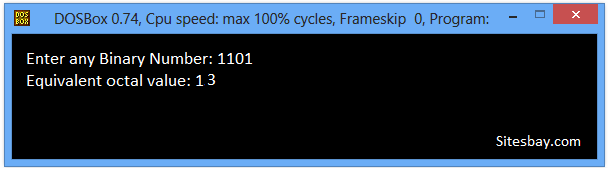# C++ Program to Convert Binary to Octal

## C++ Program to Convert Binary to Octal Number

To convert binary to octal in C++ Programming, you have to ask to the user to enter any number in binary to convert it into octal to display the equivalent value in octal on the screen.

Before write this code you must be basic kanowledge about For Loop in C++, While Loop in C++ and Modulus Operator in C++.

### Algorithom for Convert Binary to Octal Number

• Take a binary number as input.
• Divide the binary number into groups of 3 bits. For each group of 3 bits, multiply each bit with the power of 2 and add them consecutively.
• Combine the result of all groups to get the output.

## C++ Program to Convert Binary to Octal

```#include<iostream.h>
#include<conio.h>

void main()
{
long int binarynum, octalnum = 0, j = 1, remainder;
clrscr();
cout<<"Enter any Binary Number: ";
cin>>binarynum;
while (binarynum != 0)
{
remainder = binarynum % 10;
octalnum = octalnum + remainder * j;
j = j * 2;
binarynum = binarynum / 10;
}
cout<<"Equivalent octal value: " <<octalnum;
getch();
}
```

## Output

```Enter any Binary Number: 1101
Equivalent octal value: 13
```## Pure VPN Privide Lowest Price VPN Just @ \$1.65. Per Month with Non Detected IP Lowest Price Non Detected IP VPN